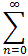#Interactive Real Analysis

Next | Previous | Glossary | Map

## 8.4. Taylor Series

### Example

Find MacLaurin series for f(x) = 1/1 + x2

The known series to start with is, of course, the Geometric series:

1/1-x =xn

for |x| < 1. If we now substitute -x2 for x we get:

1/1+x2 = 1/1-(-x2) =(-x2)n =(-1)n x2n

and the series converges to the function for |x|2 < 1, i.e. for |x| < 1.

It is interesting that the series only converges for |x| < 1 even though the function it represents is infinitely often differentiable for all x. The reason for that is that the function has a singularity at x = i, the imaginary unit, because 1 + (i)2 = 0. That singularity is invisible when we consider real numbers only, but is just on the border of a circle (of convergence) centered at zero with radius 1. Thus, this invisible imaginary singularity prevents the radius of convergence to be bigger than 1, even though there is nothing wrong with our function on the real line.

Please note that this method has the big advantage that we don't have to prove convergence and the substitution usually reveals the radius of convergence quickly. But we do need to have an idea of which series to start with, which can be quite tricky.(-1)n x2n f(x) = 1/1+x2
Next | Previous | Glossary | Map